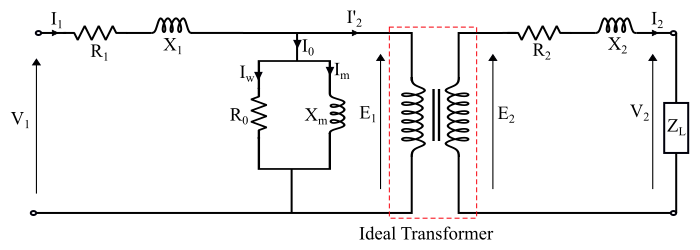# Equivalent Circuit of a Transformer Explained with Examples

## No-Load Equivalent Circuit of Transformer

The figure shows the no-load equivalent circuit of a practical transformer. In this, the practical transformer is replaced by an ideal transformer with a resistance R0 and an inductive reactance Xm in parallel with its primary winding. The resistance R0 represents the iron losses so the current IW passes it and supplies the iron losses. The inductive reactance Xm draws the magnetising current Im which produces the magnetic flux in the core.Therefore,

$$\mathrm{Iron\:losses \:of\: practical\: transformer\: = I_W^2 𝑅_{0} =\frac{𝑉_{1}^2}{𝑅_{0}}}$$

Also, from the equivalent circuit,

$$\mathrm{𝑉_{1} = 𝐼_{𝑊}𝑅_{0} = 𝐼_{𝑚}𝑋_{𝑚}}$$

The no-load current is given by phasor sum of current IW and the magnetising current Im i.e.

$$\mathrm{𝑰_{𝟎} = 𝑰_{𝑾} + 𝑰_{𝒎}}$$

## Exact Equivalent Circuit of Transformer

The exact equivalent circuit of the transformer is shown in the figure. In which, the resistance R1 is the primary winding resistance and resistance R2 is the resistance of secondary winding. Likewise, the inductive reactance X1 is the primary winding leakage reactance and the reactance X2 is the secondary winding leakage reactance. The parallel circuit R0 – Xm is the no-load equivalent circuit of the transformer.As in the exact equivalent circuit of the transformer, all the Imperfections are represented by various circuit elements. Therefore, the transformer is now an ideal one. From the exact equivalent circuit, it can be seen that there are two electrical circuit which are separated by an ideal transformer that changes the voltage and current as per the equation given below.

$$\mathrm{𝐾 =\frac{𝐸_{2}}{𝐸_{1}}=\frac{𝑁_{2}}{𝑁_{1}}=\frac{I_{2}^{\prime}}{I_{2}}}$$

Now, consider a load of Impedance ZL is connected across the secondary winding of the transformer, thus, the induced emf E2 causes a secondary current I2. Due to this I2 voltage drops occur in I2R2 and I2X2 so that the load voltage V2 will be less than E2 and is given by,

$$\mathrm{V_{2} = 𝐸_{2} − I_{2}(𝑅_{2} + 𝑗𝑋_{2}) = 𝐸_{2} − I_{2}𝑍_2}$$

Also, the total primary current (I1) drawn from the supply is equal to the phasor sum of no-load current (I0) and the current I’2 which is required to supply the load current through the secondary winding. Thus,

$$\mathrm{𝑰_{𝟏} = 𝑰_{𝟎} + I_{2}^{\prime}}$$

The primary voltage V1 is given by adding drops I1R1 and I1X1 to the emf E1 i.e.

$$\mathrm{𝑉_{1} = −𝐸_{1} + 𝐼_{1}(𝑅_1 + 𝑗𝑋_1) = −𝐸_{1} + 𝐼_{1}𝑍_1}$$

Here, the nEgative sign of E1 denotes that the E1 is 180° out of phase with V1

## Numerical Example

A 6600/11000 V, 1000 kVA transformer has the following parameters −

$$\mathrm{𝑅_{1} = 0.04 \omega; 𝑅_{2} = 0.55 \omega; 𝑅_{0} = 1500 \Omega}$$

$$\mathrm{𝑋_{1} = 0.072 \omega; 𝑋_{2} = 1.45 \omega; 𝑋_{𝑚} = 300 \Omega}$$

The transformer is supplying full-load at a power factor of 0.85 lagging. Using exact equivalent circuit, determine the input current.## Solution

Here,

$$\mathrm{Transformation\:ratio,\:𝐾 =\frac{11000}{6600} = 1.67}$$

Taking the load voltage as the reference phasor, then

$$\mathrm{V_{2} = 11000\angle0° V}$$

Now, the full load secondary current is

$$\mathrm{I_{2} =\frac{𝑘𝑉𝐴}{V_{2}}=\frac{1000 × 10^3}{11000} = 90.91 A}$$

Since the p.f. of the load is given equal to 0.85 lagging, therefore, the secondary current will be,

$$\mathrm{I_{2} = 90.91\angle − 31.78° A}$$

The Impedance of secondary winding is

$$\mathrm{𝑍_{2} = 𝑅_{2} + 𝑗𝑋_{2} = 0.55 + 𝑗1.45 = 1.55\angle69.23° \omega}$$

Thus, the secondary emf E2 is given by,

$$\mathrm{𝐸_{2} = V_{2} + I_{2}𝑍2 = (11000\angle0°) + (90.91\angle − 31.78° × 1.55\angle69.22°)}$$

$$\mathrm{⇒ 𝐸_{2} = 11000\angle0° + 140.91\angle37.44 = (11000 + 111.88 + j85.66) V}$$

$$\mathrm{⇒ 𝐸_{2} = 11111.88 + 𝑗85.66 = 11112.21\angle0.44° V}$$

Now, the primary emf E1 is given by,

$$\mathrm{𝐸_{1} =\frac{𝐸_{2}}{𝐾}=\frac{11112.21\angle0.44°}{1.67} = 6654.02\angle0.44° V}$$

Also,

$$\mathrm{I'_{2} = 𝐾I_{2} = 1.67 × 90.91\angle − 31.78°}$$

$$\mathrm{⇒ I'_{2} = 151.52\angle − 31.78° = (128.8 − 𝑗79.79) A}$$

The core-loss component of no-load current is

$$\mathrm{𝐼_{𝑊} =\frac{𝐸_{1}}{𝑅_{0}}=\frac{6654.02\angle0.44°}{1500}}$$

$$\mathrm{⇒ 𝐼_{𝑊} = 4.436\angle0.44° = (4.44 + 𝑗0.034) A}$$

And, the magnetising component of no-load current is

$$\mathrm{𝐼_{𝑚} =\frac{𝐸_{1}}{𝑋_{𝑚}}=\frac{6654.02\angle0.44°}{𝑗300}}$$

$$\mathrm{⇒ 𝐼_{𝑚} = 22.18\angle − 89.56° = (0.17 − 𝑗22.18) A}$$

$$\mathrm{𝑰_{𝟎} = 𝑰_{𝑾} + 𝑰_{𝒎} = (4.44 + 𝑗0.034) + (0.17 − 𝑗22.18)}$$
$$\mathrm{⇒ 𝐼_{0} = (4.61 − 𝑗22.15) A}$$
$$\mathrm{𝑰_{𝟏} = 𝑰'_{𝟐} + 𝑰_{𝟎} = (128.8 − 𝑗79.79) + (4.61 − 𝑗22.15)}$$
$$\mathrm{⇒ 𝐼_{1} = (133.41 − 𝑗101.94) A = 167.89\angle − 37.38° A}$$